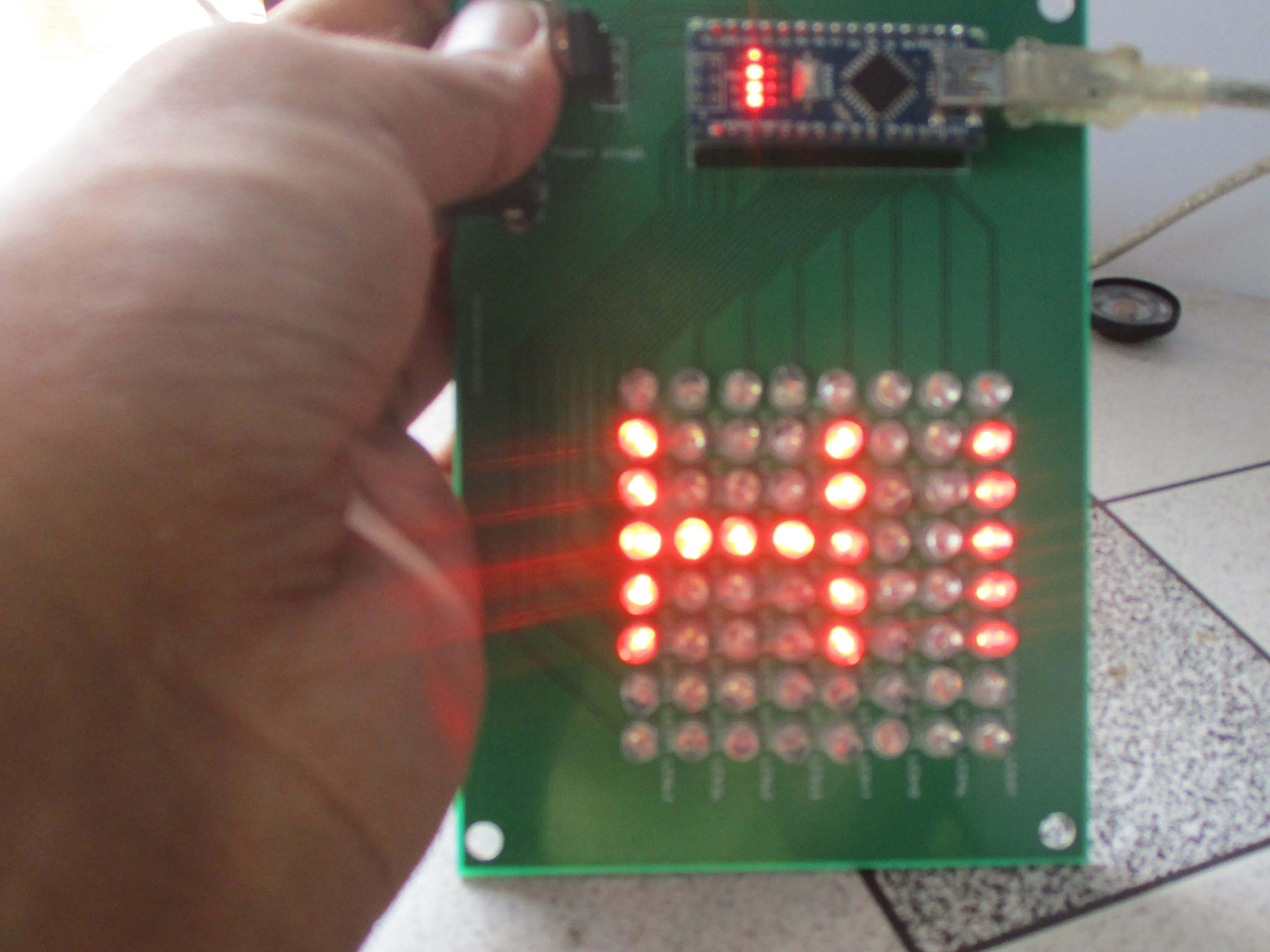# Led Matrix DIY for Arduino Nano

Led Matrix DIY for Arduino Nano

# Dot Matrix 8×8

Make a cheap and good looking LED matrix## Things used in this project

### Hardware components× 1LED (generic)
× 1× 1

### Software apps and online services### Hand tools and fabrication machinesSoldering iron (generic)

## Code

### Code

C/C++

```//RoboCircuits
//Website http://robocircuits.com

byte H[] = {
B00000000,
B10001001,
B10001001,
B11111001,
B10001001,
B10001001,
B00000000,
B00000000};

byte R[] = {
B11111100,
B10000010,
B10000001,
B10000010,
B11111100,
B10010000,
B10001000,
B10000010};

byte S[] = {
B01111111,
B10000000,
B10000000,
B10000000,
B00000000,
B10000000,
B10000000,
B01111111};

byte R[] = {
B11111100,
B10000010,
B10000001,
B10000010,
B11111100,
B10000100,
B10000010,
B10000001};

byte C[] = {
B00111111,
B01000000,
B10000000,
B10000000,
B10000000,
B10000000,
B01000000,
B00111111};

byte random1[] = {
B01010101,
B10101010,
B01010101,
B10101010,
B01010101,
B10101010,
B01010101,
B10101010};

byte random2[] = {
B10101010,
B01010101,
B10101010,
B01010101,
B10101010,
B01010101,
B10101010,
B01010101};

const int columnPins[] = { 12, 11, 10, 9, 8, 7, 6, 5};
const int rowPins[] = { 4, 3, 2, 0, 1, 13, 14, 15};
void setup() {
for (int i = 0; i < 8; i++)
{
pinMode(rowPins[i], OUTPUT); // make all the LED pins outputs
pinMode(columnPins[i], OUTPUT);
digitalWrite(columnPins[i], HIGH); // disconnect column pins from Ground
}
}

void loop()
{
int Delay = 800 ;

randomeffect();
show(H, 1000);

randomeffect();
show(R, 1000);

randomeffect();
show(C, 1000);
randomeffect();
show(H, 1000);

randomeffect();
show(R, 1000);

randomeffect();
show(C, 1000);
randomeffect();
show(H, 1000);

randomeffect();
show(R, 1000);

randomeffect();
show(C, 1000);

delay(Delay);
}

void randomeffect()
{
for(int a=0;a<2;a++)
{
show(random1,30);
delay(40);
show(random2,30);
delay(40);
}
}

void show( byte * image, unsigned long duration)
{
unsigned long start = millis();
while (start + duration > millis())
{
for(int row = 0; row < 8; row++)
{
digitalWrite(rowPins[row], HIGH); // connect row to +5 volts
for(int column = 0; column < 8; column++)
{